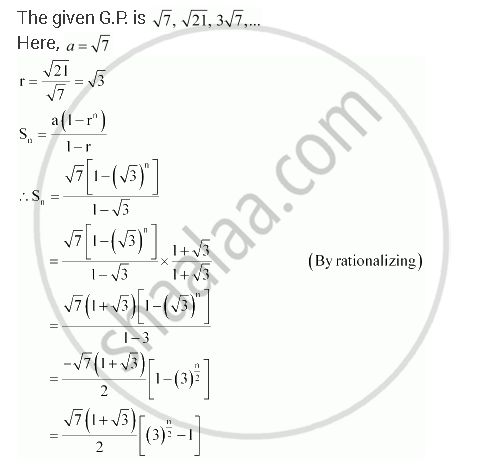CBSE (Arts) Class 11CBSE
Share
Notifications

View all notifications
Books Shortlist
Your shortlist is empty

# Find the Sum To N Terms in the Geometric Progression Sqrt7, Sqrt21,3sqrt7... - CBSE (Arts) Class 11 - Mathematics

Login
Create free account

Forgot password?
ConceptGeometric Progression (G. P.)

#### Question

Find the sum to n terms in the geometric progression sqrt7, sqrt21,3sqrt7...

#### SolutionIs there an error in this question or solution?

#### APPEARS IN

NCERT Solution for Mathematics Textbook for Class 11 (2018 to Current)
Chapter 9: Sequences and Series
Q: 8 | Page no. 192
Solution Find the Sum To N Terms in the Geometric Progression Sqrt7, Sqrt21,3sqrt7... Concept: Geometric Progression (G. P.).
S